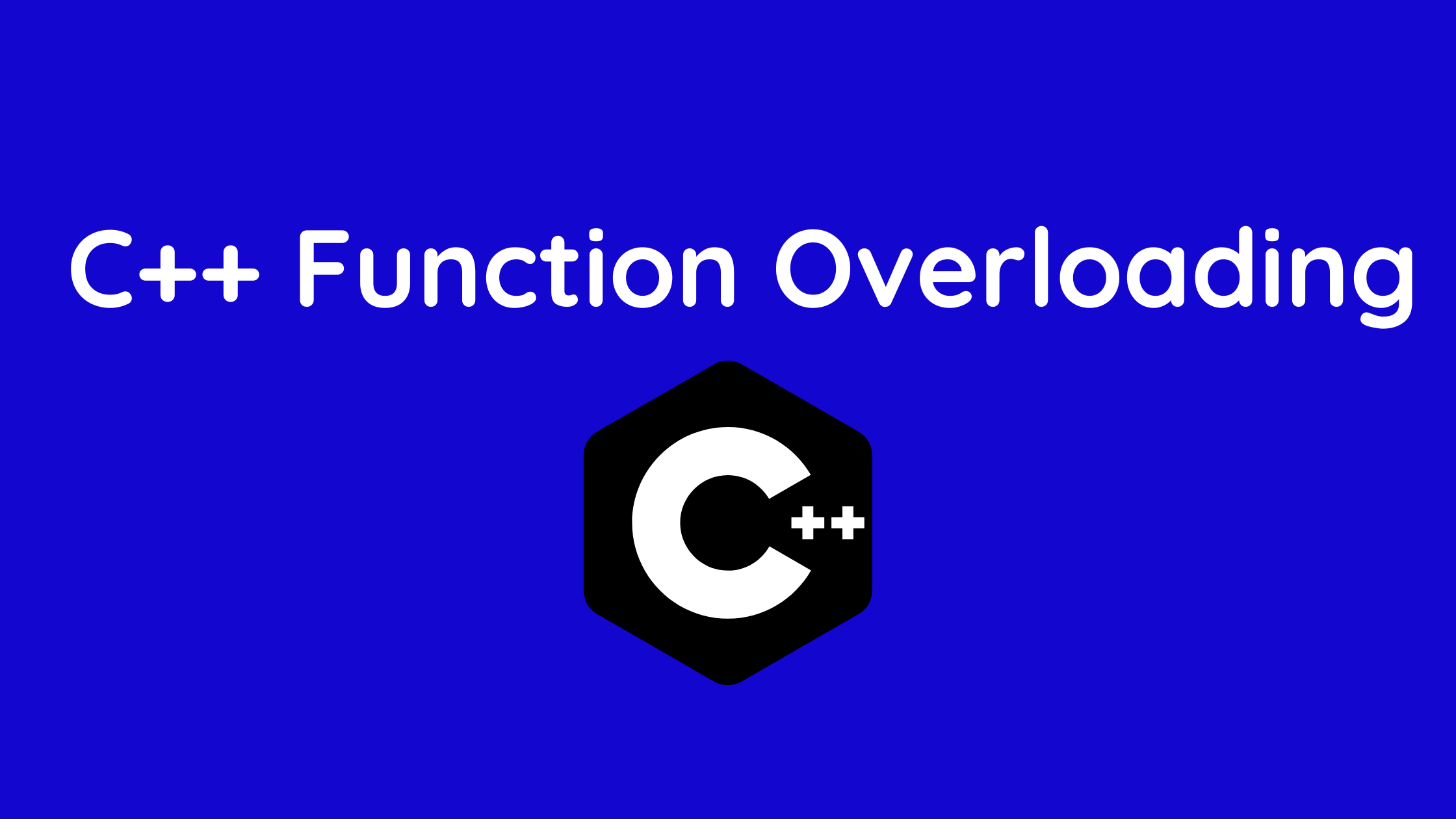The ability for two or more functions to have the same name but different parameters is known as function overloading in c++ in object-oriented programming.

• One example of a polymorphism feature in C++ is function overloading.
• Multiple functions with the same name may be specified in the same scope in C++.
• These are referred to as overloads or overloaded functions. With overloaded functions, you can provide a function with a variety of interpretations based on the types and quantities of its arguments.
• In C++, two functions with different numbers and/or types of arguments can share the same name.

Overloaded functions are those that have the same name but different arguments. For instance:

```// same name different arguments
int test() { }
int test(int a) { }
float test(double a) { }
int test(int a, double b) { }```

Here, each of the four functions is overloaded.

You’ll see that none of these 4 functions have the same return types. Different return types may or may not be included in overloaded functions, but distinct arguments are a requirement. For instance

```// Error code
int test(int a) { }
double test(int b){ }```

Both of these functions are identical in terms of name, type, and a number of parameters. The compiler will therefore produce an error.

Contents

```// Program to compute absolute value
// Works for both int and float

#include <iostream>
using namespace std;

// function with float type parameter
float absolute(float var){
if (var < 0.0)
var = -var;
return var;
}

// function with int type parameter
int absolute(int var) {
if (var < 0)
var = -var;
return var;
}

int main() {

// call function with int type parameter
cout << "Absolute value of -5 = " << absolute(-5) << endl;

// call function with float type parameter
cout << "Absolute value of 5.5 = " << absolute(5.5f) << endl;
return 0;
}```

Output

Absolute value of -5 = 5
Absolute value of 5.5 = 5.5

We overload the absolute() function in this software. The appropriate function is called depending on the kind of parameter given during the function call.

```#include <iostream>
using namespace std;

class printData {
public:
void print(int i) {
cout << "Printing int: " << i << endl;
}
void print(double  f) {
cout << "Printing float: " << f << endl;
}
void print(char* c) {
cout << "Printing character: " << c << endl;
}
};

int main(void) {
printData pd;

// Call print to print integer
pd.print(5);

// Call print to print float
pd.print(500.263);

// Call print to print character
pd.print("Hello C++");

return 0;
}
```

## Output

Printing int: 5
Printing float: 500.263
Printing character: Hello C++

```#include <iostream>
using namespace std;

// function with 2 parameters
void display(int var1, double var2) {
cout << "Integer number: " << var1;
cout << " and double number: " << var2 << endl;
}

// function with double type single parameter
void display(double var) {
cout << "Double number: " << var << endl;
}

// function with int type single parameter
void display(int var) {
cout << "Integer number: " << var << endl;
}

int main() {

int a = 5;
double b = 5.5;

// call function with int type parameter
display(a);

// call function with double type parameter
display(b);

// call function with 2 parameters
display(a, b);

return 0;
}```

Output

Integer number: 5
Float number: 5.5
Integer number: 5 and double number: 5.5

In this case, three calls to the display() function are made with various arguments. The appropriate show() function is called based on the amount and kind of arguments given.

All of these functions have the same return type, however, function overloading does not necessarily need this.

Note: A lot of the standard library functions in C++ are overloaded. The sqrt() function, for instance, accepts double, float, int, etc. as parameters. Due to C++’s overloaded sqrt() method, this is feasible.

```#include<iostream>
using namespace std;

int sum(int a, int b) {
// function with name sum and,
// returns sum of two numbers
return a + b;
}

int sum(int a, int b, int c) {
// function with name sum and,
// returns sum of three numbers
return a + b + c;
}

int main() {
// calculating sum of two numbers
int sum_1 = sum(5, 10);
cout<<"5 + 10 = "<<sum_1<<endl;

// calculating sum of three numbers
int sum_2 = sum(5, 10, 6);
cout<<"5 + 10 + 6 = "<<sum_2<<endl;

return 0;
}

```

Output

5 + 10 = 15
5 + 10 + 6 = 21

In this case, the sum() method is used twice with two separate inputs. Depending on how many parameters are supplied during the function call, the matching function sum () is invoked. Sum(), the appropriate function, is invoked.

Note: In this situation, both the sum of the overloaded functions () have the same return type, however, function overloading allows for distinct return values for the overloaded functions.

You May Also Like :

Functions in C++ with Example | C++ Programming

https://theudaipurstore.com/maharana-pratap/